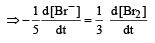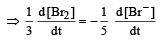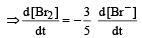Courses

# Test: Chemical Kinetics 2 - From Past 28 Years Questions

## 30 Questions MCQ Test Chemistry Class 12 | Test: Chemical Kinetics 2 - From Past 28 Years Questions

Description
This mock test of Test: Chemical Kinetics 2 - From Past 28 Years Questions for NEET helps you for every NEET entrance exam. This contains 30 Multiple Choice Questions for NEET Test: Chemical Kinetics 2 - From Past 28 Years Questions (mcq) to study with solutions a complete question bank. The solved questions answers in this Test: Chemical Kinetics 2 - From Past 28 Years Questions quiz give you a good mix of easy questions and tough questions. NEET students definitely take this Test: Chemical Kinetics 2 - From Past 28 Years Questions exercise for a better result in the exam. You can find other Test: Chemical Kinetics 2 - From Past 28 Years Questions extra questions, long questions & short questions for NEET on EduRev as well by searching above.
QUESTION: 1

### Select the rate law that corresponds to data shown for the following reaction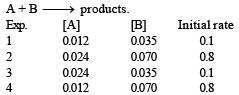Solution:

From data 1 and 3, it is clear that keeping (B) const, When [A] is doubled, rate remains unaffected. Hence rate is independent of [A]. from 1 and 4, keeping [A] constant, when [B] is doubled, rate become 8 times. Hence [rate ∝ [ B]3].

QUESTION: 2

### For an exothermic reaction, the energy of activation of the reactants is 

Solution:

Ea(Forward) + ΔH = Ea(back word)
For Exothermic reaction, ΔH = –ve and
∴  activation energy of reactant is less than the energy of activation of products.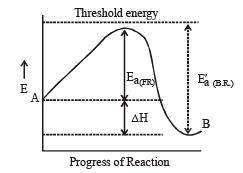QUESTION: 3

### A chemical reaction is catalyzed by a catalyst X.Hence X 

Solution:

A catalyst affects equally both forward and backward reactions, therefore it does not affect equilibrium constant of reaction.

QUESTION: 4

A substance 'A' decomposes by a first or der reaction starting initially with [A] = 2.00 m and after 200 min, [A] becomes 0.15 m. For this reaction t1/2 is 

Solution:

Given initial concentration (a) = 2.00 m; Time taken (t) = 200 min and final concentration (a – x)    = 0.15 m. For a first order reation rate constant,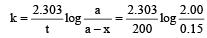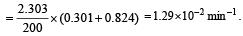Further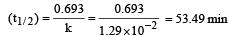QUESTION: 5

The rate of reaction depends upon the 

Solution:

The rate of a reaction is the speed at which the reactants are converted into products.
It depends upon the concentration of reactants. e.g for the reaction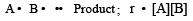QUESTION: 6

In a reversible reaction the energy of activation of the forward reaction is 50 kcal. The energy of activation for the reverse reaction will be 

Solution: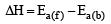Thus energy of activation for reverse reaction depend upon whether reaction is exothermic or endothermic If reaction is exothermic,  ΔH = +ve Ea(b) > Ea(f) If reaction is endothermic  ΔH =+ve Ea(b) < Ea(f )

QUESTION: 7

In a reaction, → Product, rate is doubled when the concentration of is doubled, and rate increases by a factor of 8 when the concentrations of both the reactants (and B) are doubled, rate law for the reaction can be written as

Solution:

\\

QUESTION: 8

Activation energy of a chemical reaction can be determined by 

Solution:

We know that the activation energy of chemical reaction is given by formula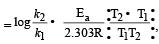where k1 is the rate constant at temperature T1 and k2 is the rate constant at temperature T2 and Ea is the activation energy.  Therefore activation energy of chemical reaction is determined by evaluating rate constant at two different temperatures.

QUESTION: 9

Half life of a first order reaction is 4 s and the initial concentration of the reactants is 0.12 M.The concentration of the reactant left after 16 s is 

Solution:

t1/2 = 4 s T = 16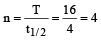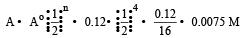where Å = initial concentration &  A = concentration left after time t

QUESTION: 10

In the following reaction , how is the rate of appearance of the underlined product related to the rate of disappearance of the underlined reactant ?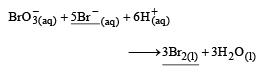Solution:

Rate of reaction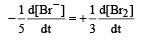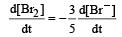QUESTION: 11

When a biochemical reaction is carried out in laboratory in the absence of enzyme then rate of reaction obtained is 10–6 times, then activation energy of reaction in the presence of enzyme is

Solution:

The presence of enzyme (catalyst) increases the speed of reaction by lowering the energy barrier, i.e. a new path is followed with lower activation energy.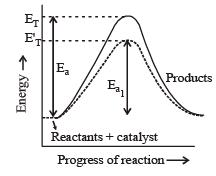Here ET is the threshold energy.
Ea and Ea1 is energy of activation of reaction in absence and presence of catalyst respectively.

QUESTION: 12

For the reaction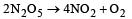rate and rate constant are 1.02 × 10–4 mol lit–1 sec–1 and 3.4 × 10–5 sec–1 respectively then concentration of N2O5 at that time will be 

Solution: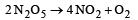from the unit of rate constant it is clear that the reaction follow first order kinetics. Hence by rate law equation,
r = k [N2O5] where r = 1.02 × 10–4,
k = 3.4 × 10–5 1.02 × 10–4 = 3.4 × 10–5 [N2O5]
[N2O5] = 3M

QUESTION: 13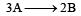, rate of reaction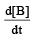is equal to

Solution:Rate of appearance of B is equal to rate of disappearance of A.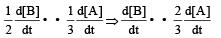QUESTION: 14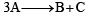, it would be a zero order reaction when

Solution:

For reactionIf it is zero order reaction r = k [A]0, i.e the rate remains same at any concentration of  'A'. i.e independent upon concentration of A.

QUESTION: 15

The temperature dependence of rate constant (k) of a chemical reaction is written in terms of Arrhenius equation,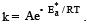. Activation energy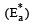of the reaction can be calculated by plotting 

Solution:

k = Ae-Ea / Rt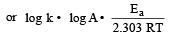Comparing the above equation with y = mx + c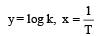Thus A plot of log10k vs 1/T should be a straight line, with slope equal to – Ea/2.303 RT and intercept equal to log A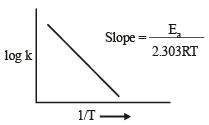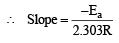or  Ea = –2.303R x Slope

QUESTION: 16

If the rate of the reaction is equal to the rate constant, the order of the reaction is 

Solution:

∵ r = k[A]n
if n = 0
r = k[A]0 or  r = k thus for zero order reactions rate is equal to the rate constant.

QUESTION: 17

The activation energy for a simple chemical reaction A → B is Ea in forward direction. The activation energy for reverse reaction 

Solution:

The activation energy of reverse reaction will depend upon whether the forward reaction is exothermic or endothermic.
As ΔH = Ea (forward reaction) – Ea(backward reaction)
For exothermic reaction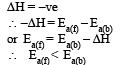for endothermic reaction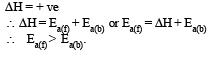QUESTION: 18

The reaction A → B follows first order kinetics.The time taken for 0.8 mole of A to produce 0.6 mole of B is 1 hour. What is the time taken for conversion of 0.9 mole of A to produce 0.675 mole of B?

Solution:

A → B For a first order reaction given a = 0.8 mol,    (a – x) = 0.8 – 0.6 = 0.2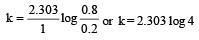again a = 0.9,   a – x = 0.9 – 0.675 = 0.225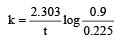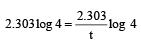Hence t = 1 hour

QUESTION: 19

The rate of a first order reaction is 1.5 × 10–2 mol L–1 min–1 at 0.5 M concentration of the reactant.The half life of the reaction is 

Solution:

For a first order reaction, A → products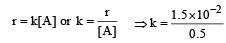= 3 × 10–2

Further,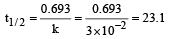QUESTION: 20

For a first order reaction A → B th e reaction rate at reactant concentration of 0.01 M is found to be 2.0 x 10-5 mol L-1 S-1. The half life period of the reaction is [2 00 5]

Solution:

Given [A] = 0.01 M
Rate = 2.0 × 10–5 mol L–1 S–1
For a first order reaction Rate = k[A]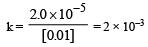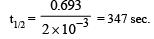QUESTION: 21

The rate of reaction between two reactants A and B decreases by a factor of 4 if the concentration of reactant B is doubled. The order of this reaction with respect to reactant B is:

Solution:

RateI  = k [A]x[B]y ... (1)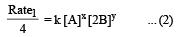or Rate1 = 4k[A]x[2B]y

From (1) and (2) we get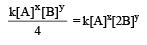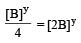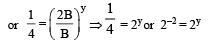or    y = –2.

QUESTION: 22

For the reaction 2A + B → 3C + D which of the following does not express the reaction rate ? 

Solution:

In the given options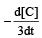will not represent the reaction rate. It should not have –ve sign as it is product. since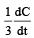show the rate of formation ofproduct C which will be positive.

QUESTION: 23

Consider the reaction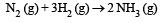The equality relationship between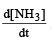and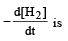Solution:

If we write rate of reaction in terms of concentration of NH3 and H2,then Rate of reaction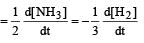So,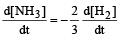QUESTION: 24

The reaction of hydrogenandiodine monochloride is given as: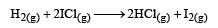The reaction is of first order with respect to H2(g) and ICI(g), following mechanisms were proposed.
Mechanism A: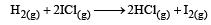Mechanism B: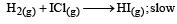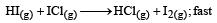Which of the above mechanism(s) can be consistent with the given information about the reaction?

Solution:

As the slowest step is the rate determining step thus the mechanism B will be more consistent with the given information also because it involve one molecule of H2 and one molecule of  ICl it can expressed as r = k [H2][ICl] Which shows that the reaction is first order w.r.t. both H2 & ICl.

QUESTION: 25

In a first-order reaction A → B, if k is rate constant and inital concentration of the reactant A is 0.5 M, then the half-life is 

Solution:

For a first order reaction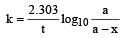when t = t½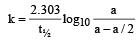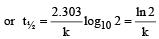QUESTION: 26

If 60% of a first order reaction was completed in 60 minutes, 50% of the same reaction would be completed in aproximately 

Solution:

For a first order reaction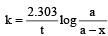when t = 60 and x = 60%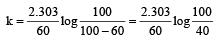Now,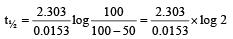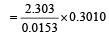=  45.31 min.

QUESTION: 27

The rate constants k1 and k2 for two different reactions are 1016 . e–2000/T and 1015 . e–1000/T, respectively. The temperature at which k1 = k2 is :

Solution:

Given,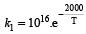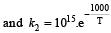when k1 and k2 are equal at any temperature T, we have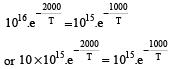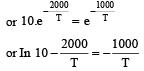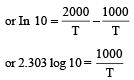or 2.303 ×1×T=1000           [∴ log 10= 1]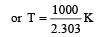QUESTION: 28

The bromination of acetone that occurs in acid solution is represented by this equation.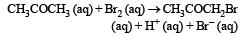These kinetic data were obtained for given reaction concentrations.

Initial Concentrations, M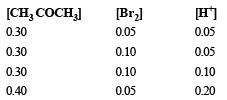Initial rate, disappearance of Br2, Ms–1

5.7×10–5
5.7 × 10–5
1.2 × 10–4
3.1 × 10–4

Base on these data, the rate equations is:

Solution:

Rewriting the given data for the reaction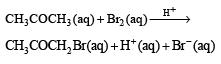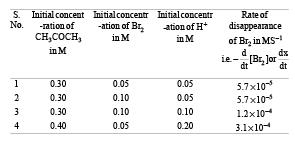Actually this reaction is autocatalyzed and involves complex calculation for concentration terms.
We can look at the above results in a simple way to find the dependence of reaction rate (i.e. rate of disappearance of Br2).
From data (1) and (2) in which concentration of CH3COCH3 and H+ remain unchanged and only the concentration of Br2 is doubled, there is no change in rate of reaction. It means the rate of reaction is independent of concentration of Br2.
Again from (2) and (3) in which (CH3CO CH3) and (Br2) remain constant but H+ increases from 0.05 M to 0.10 i.e. doubled, the rate of reaction changes from 5.7×10–5 to 1.2 × 10–4 (or 12 × 10–5), thus it also becomes almost doubled. It shows that rate of reaction is directly proportional to [H+].
From (3) and (4), the rate should have doubled due to increase in conc of [H+] from 0.10 M to 0.20 M but the rate has changed from 1.2× 10–4 to 3.1×10–4. This is due to change in concentration of CH3 CO CH3 from 0.30 M to 0.40 M. Thus the rate is directly proportional to [CH3 COCH3].
We now get rate = k [CH3COCH3]1[Br2]0[H+]1
= k [CH3COCH3][H+].

QUESTION: 29

For the reaction,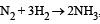,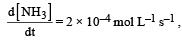the value of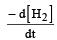would be dt:

Solution:

Rate of disappearance of H2 = rate of formation of NH3.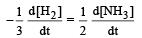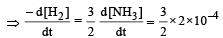= 3 ×10 –4 mol L–1s –1

QUESTION: 30

In the reaction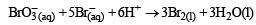The rate of appearance of bromine (Br2) is  related to rate of disappearance of bromide ions as following:

Solution:

Rate of disappearance of Br
= rate of appearance of Br2